﻿ Java数据结构专题解析之栈和队列的实现 - 自由资讯

# Java数据结构专题解析之栈和队列的实现

##### 目录
• 1. 栈
• 1.1 概念
• 1.2 助解图题
• 1.3 栈的数组实现
• 1.4 问题
• 1.5 栈的单链表实现
• 2. 队列
• 2.1 概念
• 2.2 问题
• 2.3 队列的单链表实现
• 2.4 数组实现队列
• 2.5 循环队列
• 2.6 双端队列
• 3. 栈和队列练习题
• 3.1 有效的括号
• 3.2 用队列实现栈
• 3.3 用栈实现队列
• 3.4 实现一个最小栈
• 3.5 设计循环队列

## 1. 栈

### 1.1 概念

• 栈：是一种特殊的线性表，其只允许在固定的一端进行插入和删除元素操作。
• 特点：栈中的数据元素遵循先进后出的原则，但要注意进的同时也可以出，元素不是要全部进展后才能出栈
• 栈顶：进行数据插入和删除操作的一端
• 栈底：栈顶的另一端
• 压栈：栈的插入操作就做进栈/压栈/入栈，入数据在栈顶
• 出栈：栈的删除操作就叫做出栈，出数据在栈顶

### 1.2 助解图题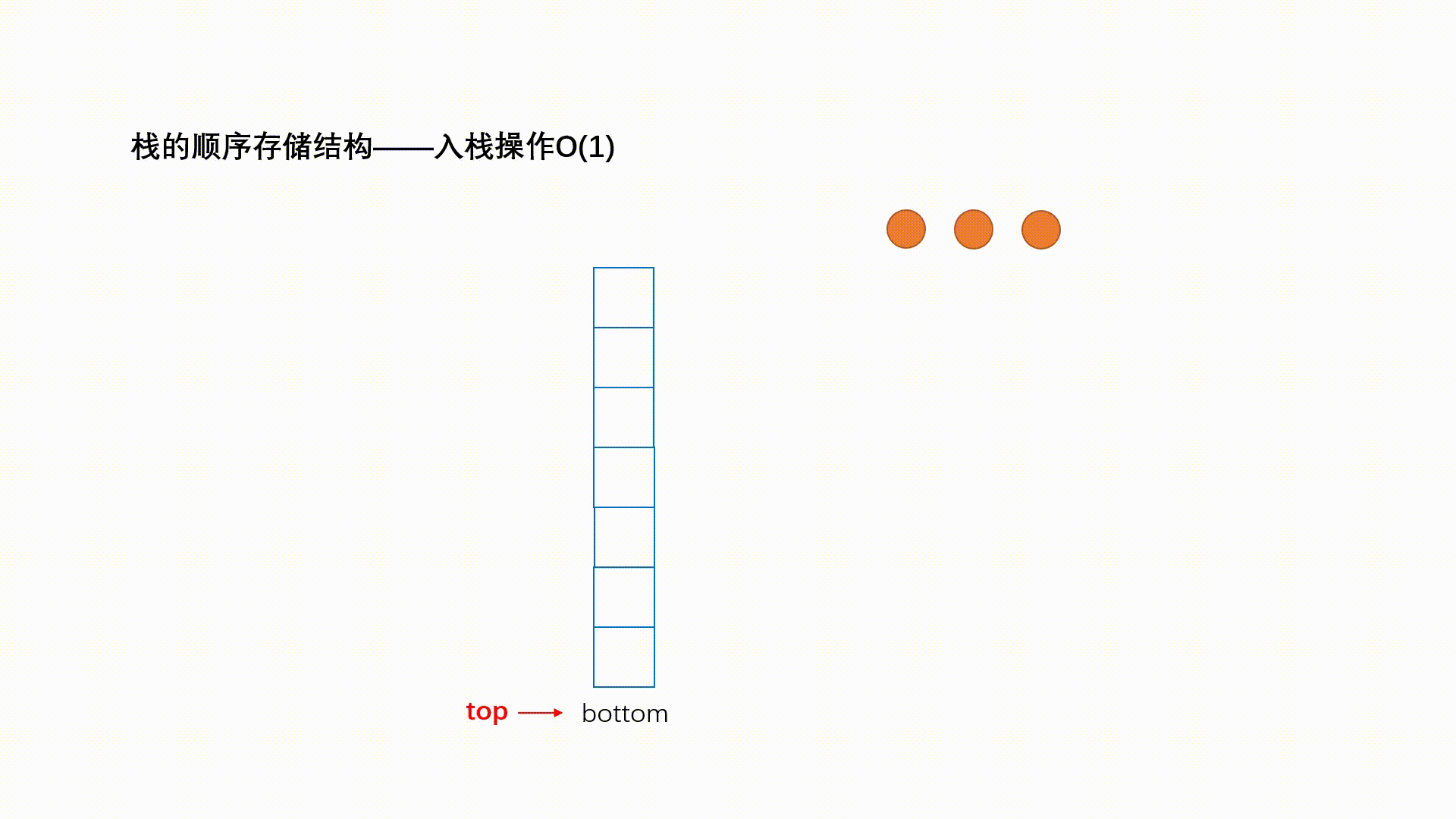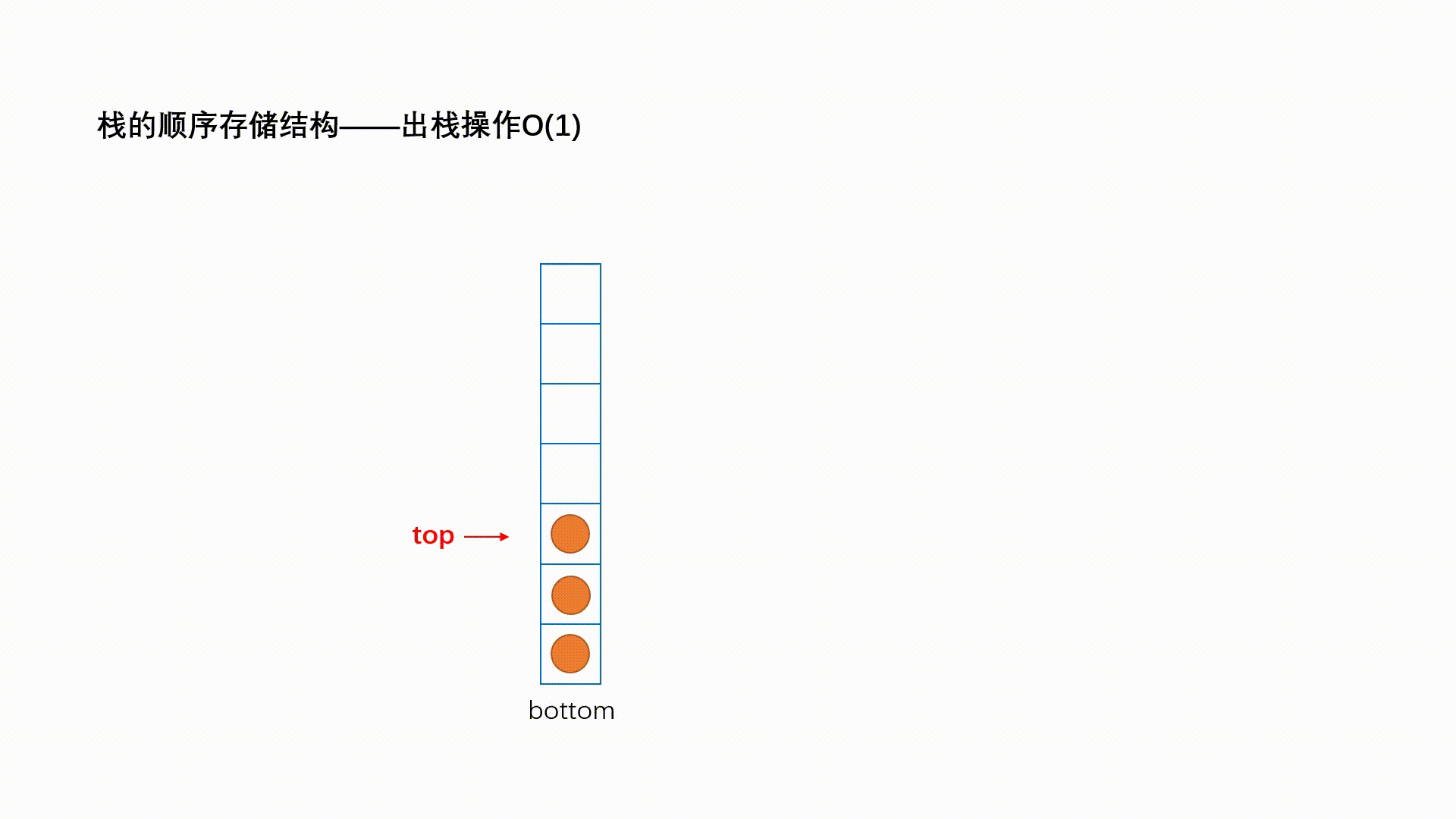• 题一： 一个栈的入栈顺序是 a、b、c、d、e，该栈不可能的出栈顺序是（ ） `A.edcba B.decba C.dceab D.abcde`

• 题二： 中缀表达式为 a + b * c + ( d * e + f ) * g，那么它的后缀表达式为什么？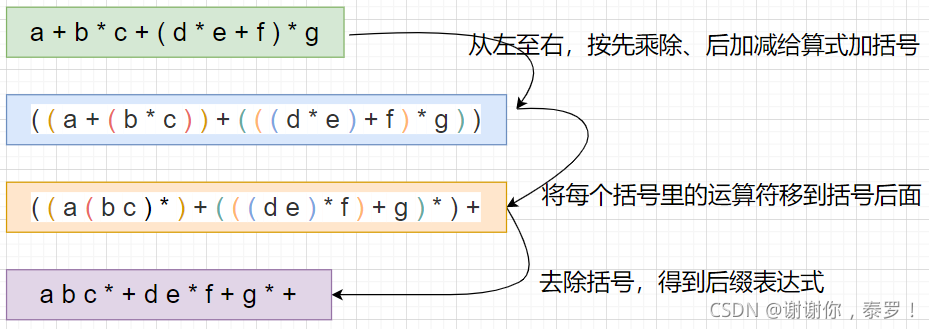• 题三： 通过栈返回后缀表达式 a b c * + d e * f + g * + 计算的结果

### 1.3 栈的数组实现

```public class MyStack{
// 定义一个整型的数组，也可以直接使用泛型
public int[] elem;
// 定义数组已经使用的长度，初始时为0
public int usedSize;
// 创建 MyStack 的构造方法
public MyStack(){
// 用 Mystack 创建对象时初始化数组的长度为10
this.elem=new int;
}
// 判断栈是否已满
public boolean isFull(){
return usedSize==this.elem.length;
}
// 压栈
public int push(int val){
if(isFull()){
// 扩容
this.elem=Arrays.copyOf(this.elem,2*this.elem.length);
}
this.elem[this.usedSize++]=val;
return val;
}
// 出栈
public int pop(){
if(empty()){
throw new RuntimeException("栈为空");
}
this.usedSize--;
return this.elem[this.usedSize];
}
// 查看栈顶元素，不删除
public int peek(){
if(empty()){
throw new RuntimeException("栈为空");
}
return this.elem[this.usedSize-1];
}
// 判断栈是否为空
public boolean empty(){
return usedSize==0;
}
}
```

### 1.4 问题

• 使用头插法：入栈时间复杂度为 O(1)，出栈时间复杂度为 O(1)
• 使用尾插法：入栈时间复杂度为 O(N)，出栈时间复杂度为 O(N)

### 1.5 栈的单链表实现

```class Node{
public int val;
public Node next;
public Node(int val){
this.val=val;
}
}
public class MyStack{
// 压栈
public int push(int val){
Node node=new Node(val);
}
// 出栈
public int pop(){
if(empty()){
throw new RuntimeException("栈为空");
}
return val;
}
// 查看栈顶元素，不删除
public int peek(){
if(empty()){
throw new RuntimeException("栈为空");
}
}
// 判断栈是否为空
public boolean empty(){
}
}
```

## 2. 队列

### 2.1 概念

• 队列：只允许在一端进行插入数据操作，在另一端进行删除操作的特殊线性表。
• 特点：队列具有先进先出的特点
• 对头：进行删除操作的一端
• 队尾：进行插入操作的一段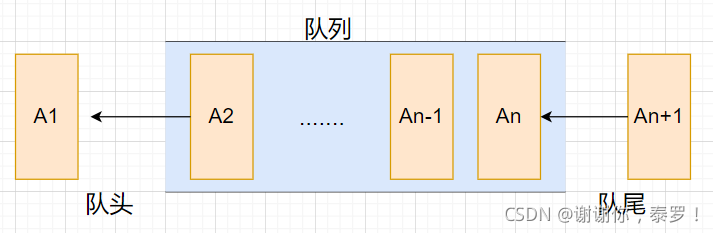### 2.2 问题

• 对于双向链表：我们只要在尾节点进行入队操作，在头节点进行出队操作就很容易实现
• 对于单链表：我们只要增加一个尾巴节点，然后尾巴节点进行入队操作，头节点进行出队操作也能实现

### 2.3 队列的单链表实现

```class Node{
public int val;
public Node next;
public Node(int val){
this.val=val;
}
}
private Node front;
private Node rear;
private int usedSize;
// 入队列
public void offer(int val){
Node node=new Node(val);
if(this.front==null){
this.front=node;
this.rear=node;
}else{
this.rear.next=node;
this.rear=node;
}
this.usedSize++;
}
// 出队列
public int poll(){
if(isEmpty()){
throw new RuntimeException("队列为空");
}
int val=this.front.val;
if(this.front.next==null){
this.front=null;
this.rear=null;
}else{
this.front=this.front.next;
}
this.usedSize--;
return val;
}
// 得到队头元素不删除
public int peek(){
if(isEmpty()){
throw new RuntimeException("队列为空");
}else{
return this.front.val;
}
}
// 判断队列是否为空
public boolean isEmpty(){
return this.usedSize==0;
}
// 得到队列长度
public int size(){
return this.usedSize;
}
}
```

### 2.4 数组实现队列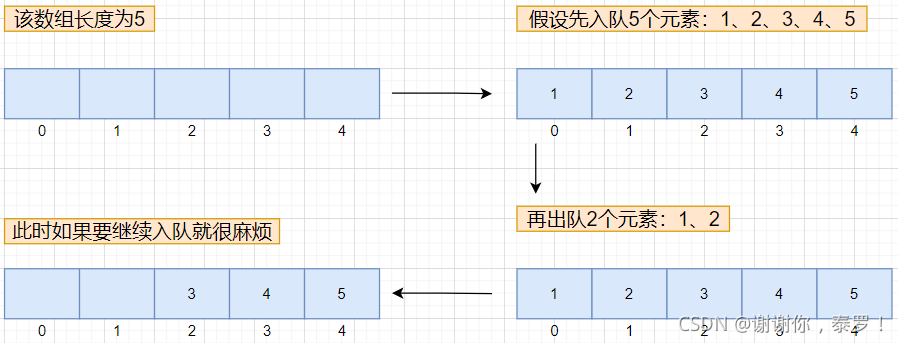• 方法一：出队时，移动数组将后面的元素往前覆盖（时间复杂度为 O(N)）
• 方法二：使用循环的方法，实现循环队列（时间复杂度为 O(1)）

### 2.5 循环队列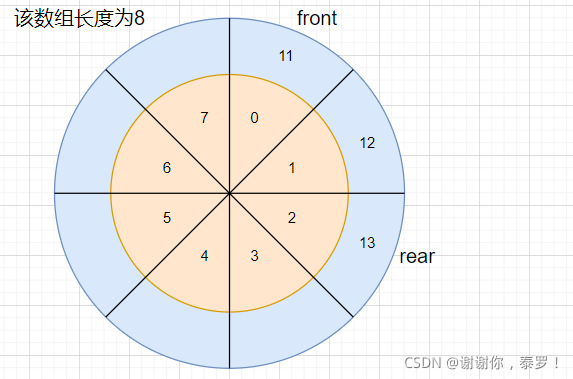• front：指向的位置就是队列的头，既已经存放数据的第一个下标（删除数据一端）
• rear：指向的位置就是队列的尾巴，即可以存放数据的第一个下标（插入数据一端）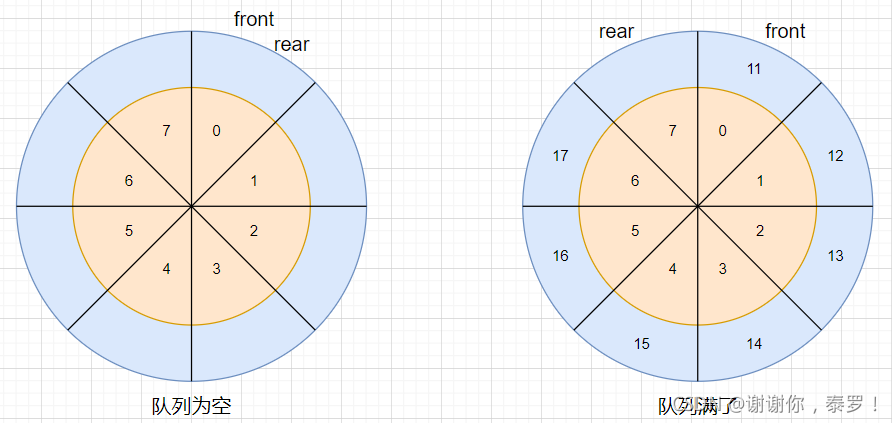`(下标+1)%数组长度`

```public class MyCircularQueue {
private int[] elem;
private int front;
private int rear;
public MyCircularQueue(int k){
this.elem=new int[k+1];
}
// 判断队列是否满了
public boolean isFull(){
return (this.rear+1)%this.elem.length==this.front;
}
// 判断队列是否为空
public boolean isEmpty(){
return this.front==this.rear;
}
// 入队
public void enQueue(int val){
if(isFull()){
this.elem= Arrays.copyOf(this.elem,2*this.elem.length);
}
this.elem[this.rear]=val;
this.rear=(this.rear+1)%this.elem.length;
}
// 出队
public int deQueue(){
if(isEmpty()){
throw new RuntimeException("队列为空");
}
int val=this.elem[this.front];
this.front=(this.front+1)%this.elem.length;
return val;
}
// 得到队头元素
public int getFront(){
if(isEmpty()){
throw new RuntimeException("队列为空");
}
return this.elem[this.front];
}
// 得到队尾元素
public int getRear(){
if(isEmpty()){
throw new RuntimeException("队列为空");
}
if(this.rear==0){
return this.elem[this.elem.length-1];
}
return this.elem[this.rear - 1];
}
}
```

### 2.6 双端队列

• 双端队列：是指允许两端都可以进行入队和出队操作的队列
• 特点：元素可以从队头出队和入队，也可以从队尾出队和入队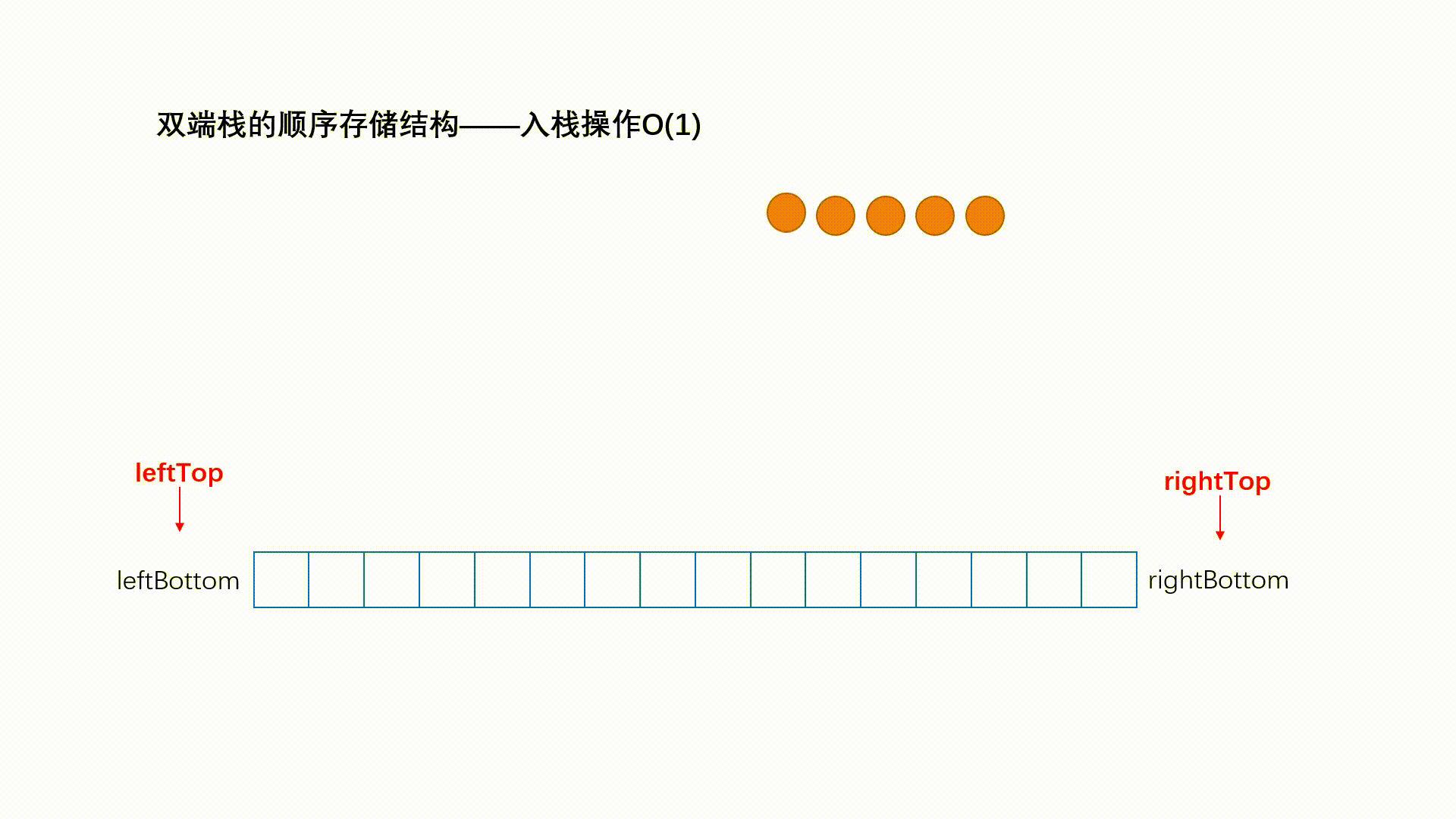## 3. 栈和队列练习题

### 3.1 有效的括号

```public boolean isValid(String s) {
Stack<Character> stack=new Stack<>();
for(int i=0;i<s.length();i++){
char ch=s.charAt(i);
switch(ch){
case ')':
if(stack.empty()||stack.pop()!='('){
return false;
}
break;
case '}':
if(stack.empty()||stack.pop()!='{'){
return false;
}
break;
case ']':
if(stack.empty()||stack.pop()!='['){
return false;
}
break;
default:
stack.push(ch);
break;
}
}
if(stack.empty()){
return true;
}
return false;
}
```

### 3.2 用队列实现栈

```class MyStack {
private Queue<Integer> q1;
private Queue<Integer> q2;
public MyStack() {
}
// 入栈
public void push(int x) {
if(!q1.isEmpty()){
q1.offer(x);
}else if(!q2.isEmpty()){
q2.offer(x);
}else{
q1.offer(x);
}
}
// 出栈
public int pop() {
if(empty()){
throw new RuntimeException("栈为空");
}
if(!q1.isEmpty()){
int val1=0;
while(!q1.isEmpty()){
val1=q1.poll();
if(!q1.isEmpty()){
q2.offer(val1);
}
}
return val1;
}else{
int val2=0;
while(!q2.isEmpty()){
val2=q2.poll();
if(!q2.isEmpty()){
q1.offer(val2);
}
}
return val2;
}
}
// 得到栈顶元素不删除
public int top() {
if(empty()){
throw new RuntimeException("栈为空");
}
if(!q1.isEmpty()){
int val1=0;
while(!q1.isEmpty()){
val1=q1.poll();
q2.offer(val1);
}
return val1;
}else{
int val2=0;
while(!q2.isEmpty()){
val2=q2.poll();
q1.offer(val2);
}
return val2;
}
}
// 判断栈是否为空
public boolean empty() {
return q1.isEmpty()&&q2.isEmpty();
}
}
```

### 3.3 用栈实现队列

```class MyQueue {
private Stack<Integer> s1;
private Stack<Integer> s2;
public MyQueue() {
s1=new Stack<>();
s2=new Stack<>();
}
// 入队
public void push(int x) {
s1.push(x);
}
// 出队
public int pop() {
if(empty()){
return -1;
}
if(s2.empty()){
while(!s1.empty()){
s2.push(s1.pop());
}
}
return s2.pop();
}
// 返回队首元素，不删除
public int peek() {
if(empty()){
return -1;
}
if(s2.empty()){
while(!s1.empty()){
s2.push(s1.pop());
}
}
return s2.peek();
}
// 判断队列是否为空
public boolean empty() {
return s1.empty()&&s2.empty();
}
}
```

### 3.4 实现一个最小栈

```class MinStack {
private Stack<Integer> stack;
private Stack<Integer> minStack;
public MinStack() {
stack=new Stack<>();
minStack=new Stack<>();
}
// 入栈
public void push(int val) {
stack.push(val);
if(minStack.empty()){
minStack.push(val);
}else{
if(val<=minStack.peek()){
minStack.push(val);
}
}
}
// 出栈
public void pop() {
int val=stack.pop();
if(minStack.peek()==val){
minStack.pop();
}
}
// 得到栈顶元素，不删除
public int top() {
return stack.peek();
}
// 得到此时栈的最小值
public int getMin() {
return minStack.peek();
}
}
```

### 3.5 设计循环队列

```class MyCircularQueue {
private int[] elem;
private int front;
private int rear;
public MyCircularQueue(int k) {
this.elem=new int[k+1];
}
// 入队列
public boolean enQueue(int value) {
if(isFull()){
return false;
}
this.elem[rear]=value;
this.rear=(this.rear+1)%this.elem.length;
return true;
}
// 出队列
public boolean deQueue() {
if(isEmpty()){
return false;
}
this.front=(this.front+1)%this.elem.length;
return true;
}
// 得到队首元素，不删除
public int Front() {
if(isEmpty()){
return -1;
}
return this.elem[front];
}
// 得到队尾元素，不删除
public int Rear() {
if(isEmpty()){
return -1;
}
if(this.rear==0){
return this.elem[this.elem.length-1];
}
return this.elem[this.rear-1];
}
// 判断队列是否为空
public boolean isEmpty() {
return this.rear==this.front;
}
// 判断队列是否满了
public boolean isFull() {
return (this.rear+1)%this.elem.length==this.front;
}
}
```

﻿